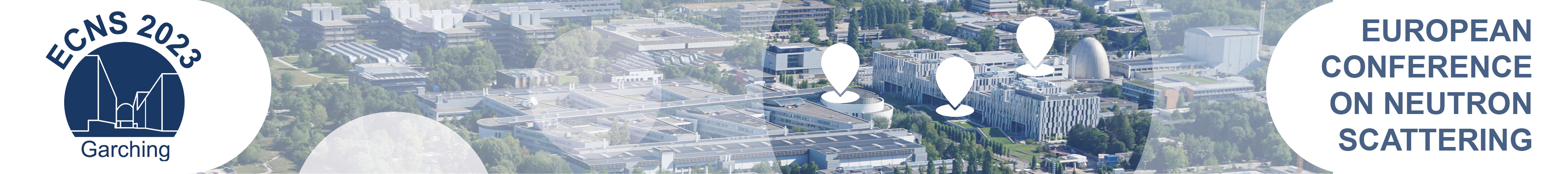#European Conference on Neutron Scattering 2023

Mar 20 – 23, 2023
Campus Garching
Europe/Berlin timezone

## Neutron diffraction study of the 1/2 quantum magnetization plateau compound Ni$_2$V$_2$O$_7$

Mar 21, 2023, 4:00 PM
2h
Yards 4 - 6 (Fakultät für Maschinenwesen)

### Yards 4 - 6

#### Fakultät für Maschinenwesen

Board: TU-148
Poster Magnetism, Superconductivity, Topological Systems, Magnetic Thin Films an other electronic phenomena

### Speakers

Dr Masashi Matsuo (National Institute for Materials Science) Yukari Matsuo (Hosei university)

### Description

We report on the results of powder neutron diffraction of Ni$_2$V$_2$O$_7$. Two crystallographic Ni$^{2+}$-ion sites carrying spin-1 exist. Magnetic phase transitions occur at $T_{\rm N1} = 6.7$ and $T_{\rm N2} = 5.7$ K. A 1/2 quantum magnetization plateau appears between 8 and 30 T at 2 K.
We carried out neutron diffraction experiments at 0 T using the HRPT diffractometer at PSI. The wavelength of the neutrons was 2.450 Å. We observed magnetic reflections at 2.3 and 6.0 K. We can index the magnetic reflections with the propagation vector $(k_x \sim 0.46, 0, 0)$, indicating an incommensurate magnetic structure. We are analyzing the data to determine the magnetic structure.
We conducted neutron diffraction experiments at zero and finite magnetic fields using the WOMBAT diffractometer at ANSTO. The wavelength of the neutrons was 2.412 Å. We observed magnetic reflections at 10 T and 1.9 K where the 1/2 quantum magnetization plateau appears. Indices of the magnetic reflections except for those at $Q = 1.06$ and $1.02$ Å$^{-1}$ are integers and nuclear reflections exist at the positions of the magnetic reflections with the integer indices. The magnetic reflections remain at 10 T and 15 K that is much higher than $T_{\rm N1}=6.7$ K, indicating that the magnetic reflections are generated by field-induced magnetic moments. The sum of $m_1$ and $m_2$ at 10 T and 1.9 K should be 2.2 because of the 1/2 quantum magnetization plateau phase and $g=2.2$. Here, $m_1$ and $m_2$ are magnitudes of field-induced magnetic moments on Ni1 and Ni2 sites, respectively. We calculated the integrated intensities of the magnetic reflections under the condition that $m_1 + m_2 = 2.2$. The calculated and experimental intensities agree with each other in the vicinity of $m_1 = 0.2$.
The magnetic reflections at $Q = 1.06$ and $1.02$ Å$^{-1}$ exist below 10 T at 1.9 K, whereas they do not exist at 10 T and 10 K. Therefore, the origin of the magnetic reflections seems to be magnetic order. Although the 1/2 quantum magnetization plateau phase at 10 T and 1.9 K is paramagnetic, the magnetic order phase may coexist.

### Primary author

Yukari Matsuo (Hosei university)

### Co-authors

Dr Andreas Dönni (National Institute for Materials Science) Dr James Hester (ANSTO) Dr Kirrily Rule (ANSTO) Dr Masashi Matsuo (National Institute for Materials Science) Dr Noriki Terada (National Institute for Materials Science) Dr Vladimir Pomjakushin (PSI)

### Presentation materials

There are no materials yet.